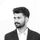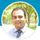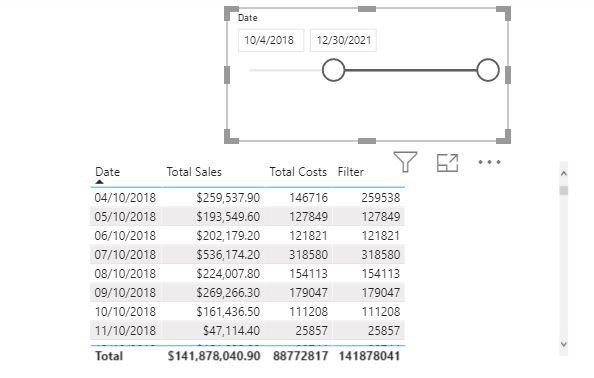cancel
Showing results for
Did you mean:Anonymous
Not applicable

## Last Year dynamically calculated

Hi there, I'm facing with the following problem.

I need to create an Last Year measure.

then I created a calculated group like this:

v_dim_time and v_fact_sales are related to 1:n

var DayToCompare = today()
var DateLastYear = EDATE(DayToCompare;-12)
return
calculate(
SELECTEDMEASURE ();
year(v_DIM_TIME[CALENDAR_DATE_SQL]) = Year(DateLastYear);
v_DIM_TIME[CALENDAR_DATE_SQL] <= dateLastYear
)

My problem is how can I to made the formula dynamical, saying for example that ytd will be evaluated at today - 365 if no other selection are made by time slicer otherview keeping the value selected ?

1 ACCEPTED SOLUTIONCommunity Champion

@Anonymous

Using ISFILTERED()  function you can identify if the column is filtered or not. Based on that, you can switch your logic.

Appreciate with a kudos
🙂

2 REPLIES 2Community Champion

Hi @Anonymous ,

Can you please share the sample data and expected output.

Have calculated values where the column changes dynamically i.e shows Total Cost incase Filter in not selected and shows Total Sales if the filter is selected.

The Date Slicer is from the Calendar Table.

Filter =
var __mini = CALCULATE ( MIN ( Dates[Date] ), ALLSELECTED ( Dates[Date] ) )
var __maxi = CALCULATE ( MAX ( Dates[Date] ), ALLSELECTED ( Dates[Date] ) )
var __mind = MIN(Dates[Date])
var __maxd = MAX(Dates[Date])
var __datediffmin = DATEDIFF(__mind,__mini,DAY)
var __datediffmax = DATEDIFF(__maxd,__maxi,DAY)
return
IF ((__datediffmin = 0 || __datediffmax = 0),[Total Sales],[Total Costs])

You can replace the Total Sales and Total Cost with the YTD and YTD from today.Let me know if this is what you are looking for.

Regards,
Harsh NathaniCommunity Champion

@Anonymous

Using ISFILTERED()  function you can identify if the column is filtered or not. Based on that, you can switch your logic.